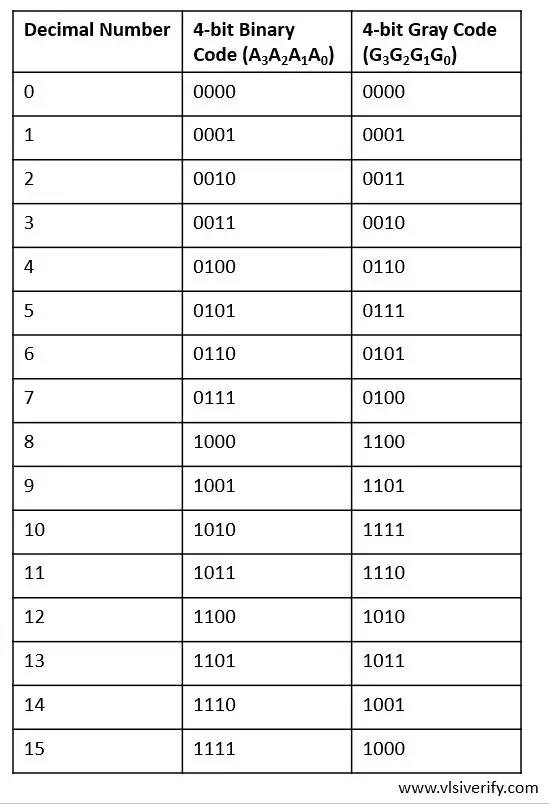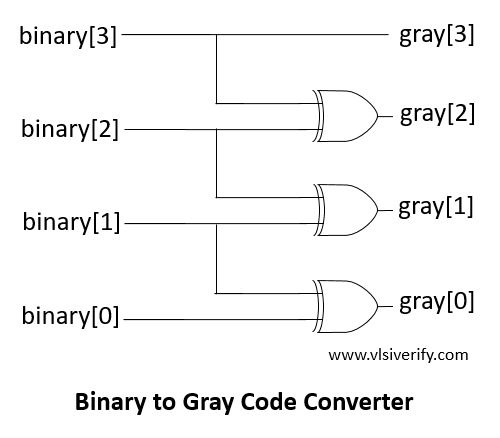Tutorials

Gray Code is similar to binary code except its successive number differs by only a single bit. Hence, it has importance in communication systems as it minimizes error occurrence. They are also useful in rotary, optical encoders, data acquisition systems, etc.

Let’s see binary numbers and their equivalent gray code in the below table.

## Truth TableBy solving using K-map technique, a simple combinational circuit turned out as## Binary to Gray Code Converter Verilog Code

``````module b2g_converter #(parameter WIDTH=4) (input [WIDTH-1:0] binary, output [WIDTH-1:0] gray);
genvar i;
generate
for(i=0;i<WIDTH-1;i++) begin
assign gray[i] = binary[i] ^ binary[i+1];
end
endgenerate

assign gray[WIDTH-1] = binary[WIDTH-1];
endmodule``````

### Testbench Code

``````module TB;
reg [3:0] binary, gray;
b2g_converter b2g(binary, gray);

initial begin
\$monitor("Binary = %b --> Gray = %b", binary, gray);
binary = 4'b1011; #1;
binary = 4'b0111; #1;
binary = 4'b0101; #1;
binary = 4'b1100; #1;
binary = 4'b1111;
end
endmodule``````

Output:

``````Binary = 1011 --> Gray = 1110
Binary = 0111 --> Gray = 0100
Binary = 0101 --> Gray = 0111
Binary = 1100 --> Gray = 1010
Binary = 1111 --> Gray = 1000``````

Compact implementation is mentioned below:

### Alternative Verilog implementation

``````module b2g_converter #(parameter WIDTH=4) (input [WIDTH-1:0] binary, output [WIDTH-1:0] gray);
assign gray = binary ^ (binary >> 1);
endmodule``````

Verilog Codes